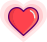1

### 参考代码

public int plus(int a, int b) {    int aTemp = 0, bTemp = 0;    while (b != 0) {        aTemp = a ^ b;        bTemp = (a & b) << 1;        a = aTemp;        b = bTemp;    }    return a;}

02

### 参考代码

public void swap(int a, int b) {    a ^= b; // a 中存放两数互异的点位    b ^= a; // 取反 b 中不同于 a 的点位，也就是实现了 b = a    a ^= b; // 取反 a 中不同于 b 的点位，也就是实现了 a = b}

03

### 参考代码

public int convertA2B(int A, int B) {    int n = A ^ B;    int count = 0;    while (n != 0) {        n = n & (n - 1); // n - 1 是将 n 的最低位为零        count++;    }    return count;}

04

LeetCode 第 136 号问题：给定一个非空整数数组，除了某个元素只出现一次以外，其余每个元素均出现两次。找出那个只出现了一次的元素。

### 参考代码

public int findOnce(int[] arr) {    int result = 0;    for (int i = 0; i < arr.length; ++i) {        result ^= arr[i];    }    return result;}

05

LeetCode 第 137  号问题：给定一个非空整数数组，除了某个元素只出现一次以外，其余每个元素均出现了三次。找出那个只出现了一次的元素。

### 参考代码

public int singleNumber(int[] nums) {    int ones = 0, twos = 0;    for (int i = 0; i < nums.length; ++i) {        // 找出当前的 3n + 1        ones = (nums[i] ^ ones) & (~twos);        // 找出当前的 3n + 2        twos = (nums[i] ^ twos) & (~ones);    }    return ones;}

06

LeetCode 第 260  号问题：给定一个整数数组 nums，其中恰好有两个元素只出现一次，其余所有元素均出现两次。找出只出现一次的那两个元素。

### 参考代码

public int[] singleNumber(int[] nums) {    if (nums == null || nums.length == 0) {        return new int;    }    int different = 0;    for (int i : nums) {        different ^= i;    }    different &= -different;    int[] ans = {0, 0};    for (int i : nums) {        if ((different & i) == 0) {            ans ^= i;        } else {            ans ^= i;        }    }    return ans;}

##原 创 热 文 推 荐